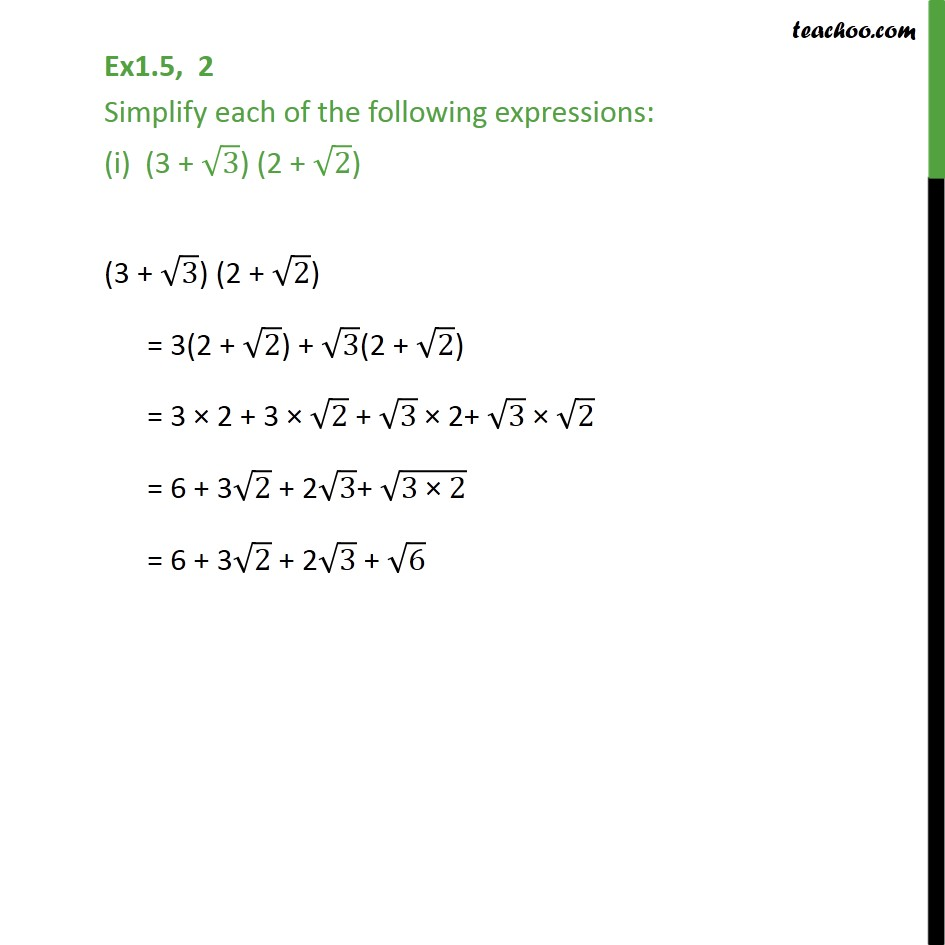Chapter 1 Class 9 Number Systems

Class 9
Important Questions for Exam - Class 9Learn in your speed, with individual attention - Teachoo Maths 1-on-1 Class

### Transcript

Ex1.5, 2 Simplify each of the following expressions: (i) (3 + 3) (2 + 2) (3 + 3) (2 + 2) = 3(2 + 2) + 3(2 + 2) = 3 2 + 3 2 + 3 2+ 3 2 = 6 + 3 2 + 2 3+ (3" " 2) = 6 + 3 2 + 2 3 + 6